Скачать презентацию Unit 6 Sampling Distributions and Statistical Inference —

874ae95af8313ae1a27791e268f1422d.ppt

• Количество слайдов: 76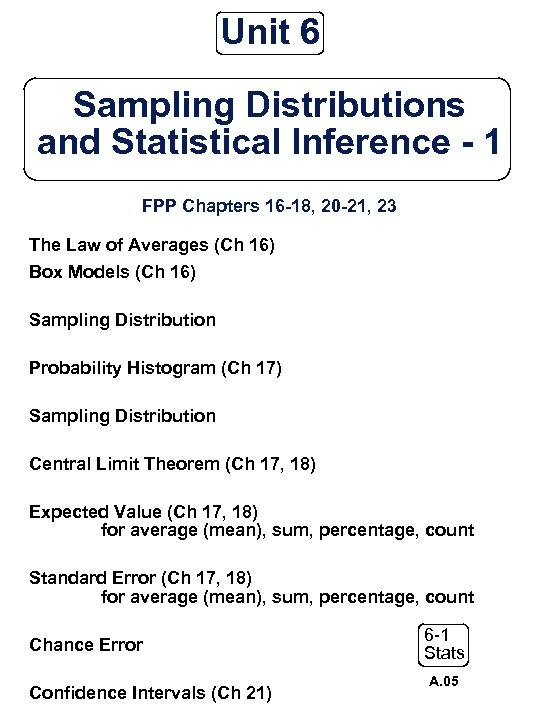Unit 6 Sampling Distributions and Statistical Inference - 1 FPP Chapters 16 -18, 20 -21, 23 The Law of Averages (Ch 16) Box Models (Ch 16) Sampling Distribution Probability Histogram (Ch 17) Sampling Distribution Central Limit Theorem (Ch 17, 18) Expected Value (Ch 17, 18) for average (mean), sum, percentage, count Standard Error (Ch 17, 18) for average (mean), sum, percentage, count Chance Error Confidence Intervals (Ch 21) 6 -1 Stats A. 05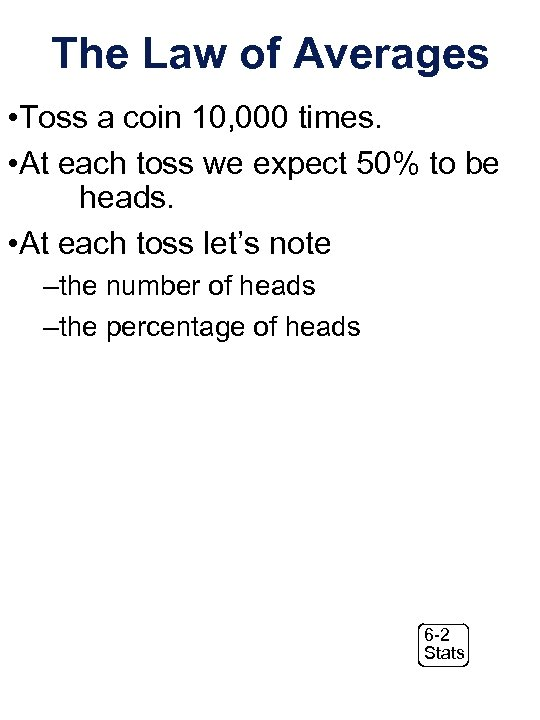The Law of Averages • Toss a coin 10, 000 times. • At each toss we expect 50% to be heads. • At each toss let’s note –the number of heads –the percentage of heads 6 -2 StatsNumber of heads 6 -3 StatsPercentage of heads 6 -4 Stats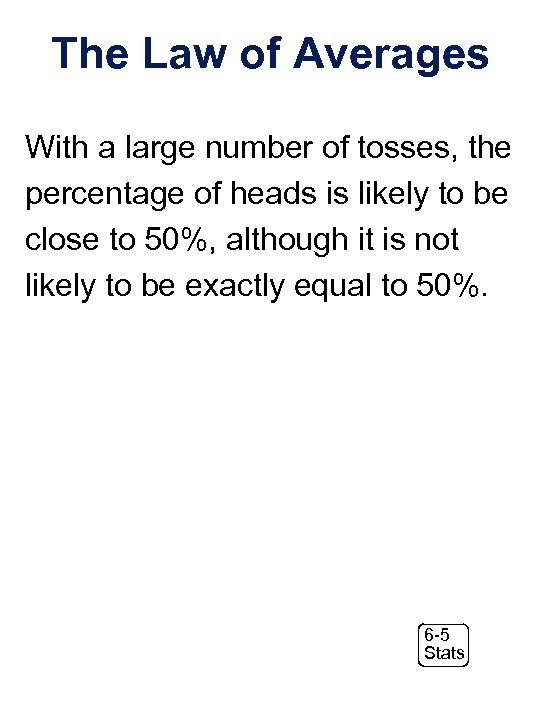The Law of Averages With a large number of tosses, the percentage of heads is likely to be close to 50%, although it is not likely to be exactly equal to 50%. 6 -5 Stats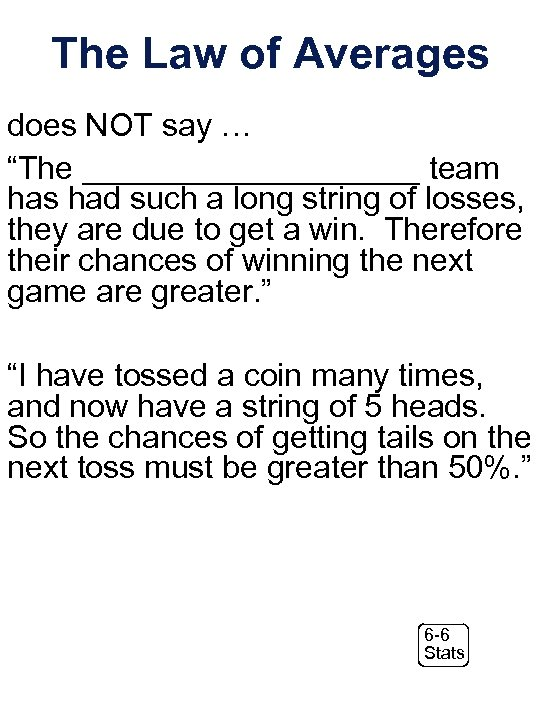The Law of Averages does NOT say … “The __________ team has had such a long string of losses, they are due to get a win. Therefore their chances of winning the next game are greater. ” “I have tossed a coin many times, and now have a string of 5 heads. So the chances of getting tails on the next toss must be greater than 50%. ” 6 -6 Stats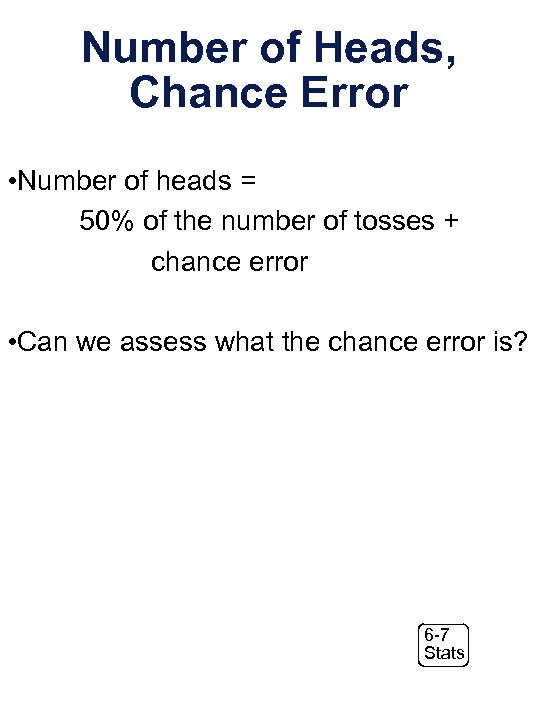Number of Heads, Chance Error • Number of heads = 50% of the number of tosses + chance error • Can we assess what the chance error is? 6 -7 Stats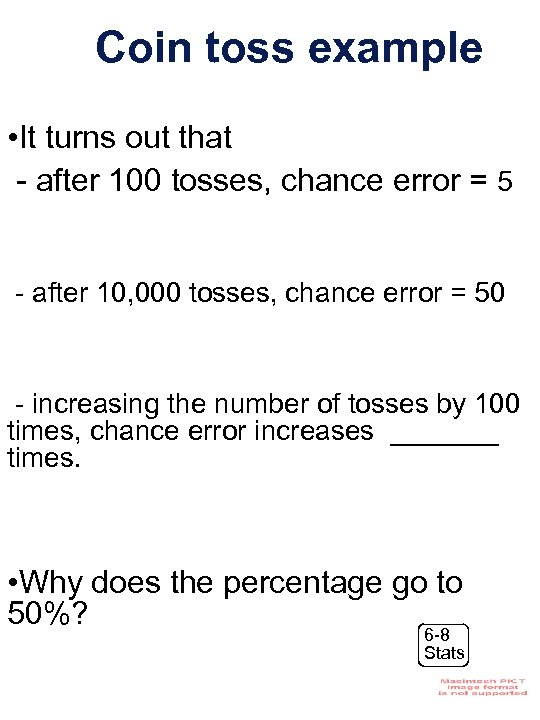Coin toss example • It turns out that - after 100 tosses, chance error = 5 - after 10, 000 tosses, chance error = 50 - increasing the number of tosses by 100 times, chance error increases _______ times. • Why does the percentage go to 50%? 6 -8 Stats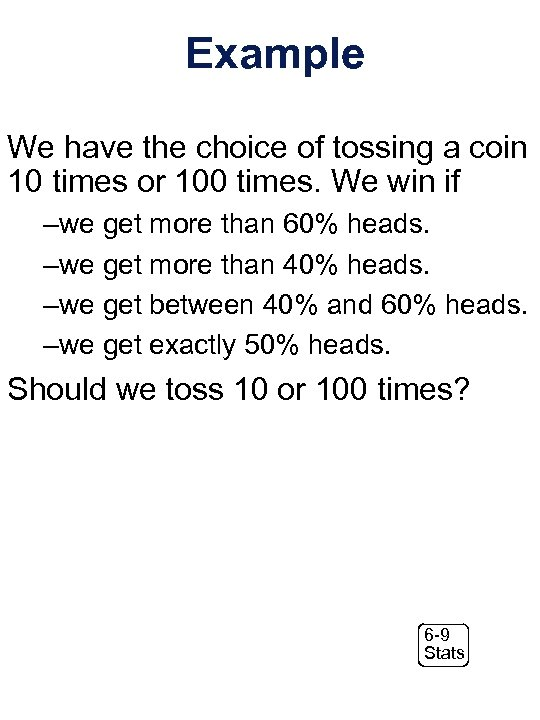Example We have the choice of tossing a coin 10 times or 100 times. We win if –we get more than 60% heads. –we get more than 40% heads. –we get between 40% and 60% heads. –we get exactly 50% heads. Should we toss 10 or 100 times? 6 -9 Stats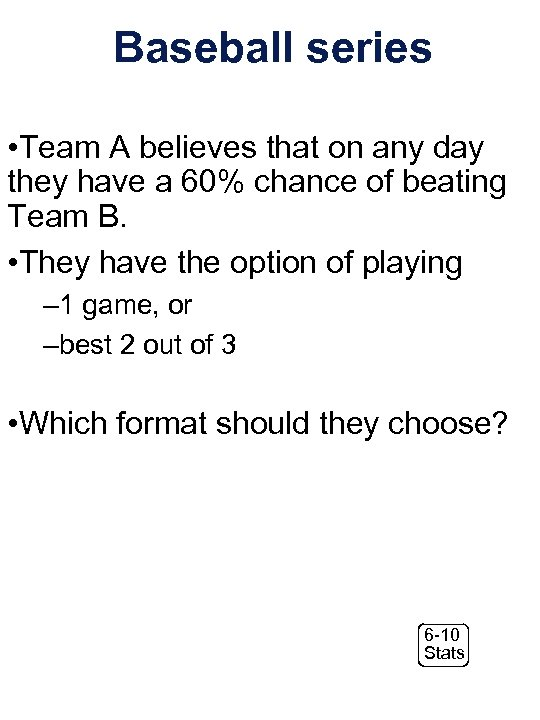Baseball series • Team A believes that on any day they have a 60% chance of beating Team B. • They have the option of playing – 1 game, or –best 2 out of 3 • Which format should they choose? 6 -10 Stats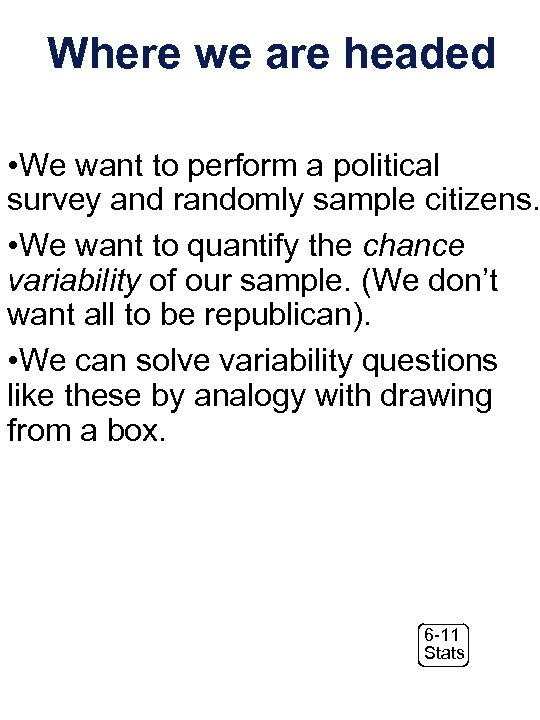Where we are headed • We want to perform a political survey and randomly sample citizens. • We want to quantify the chance variability of our sample. (We don’t want all to be republican). • We can solve variability questions like these by analogy with drawing from a box. 6 -11 Stats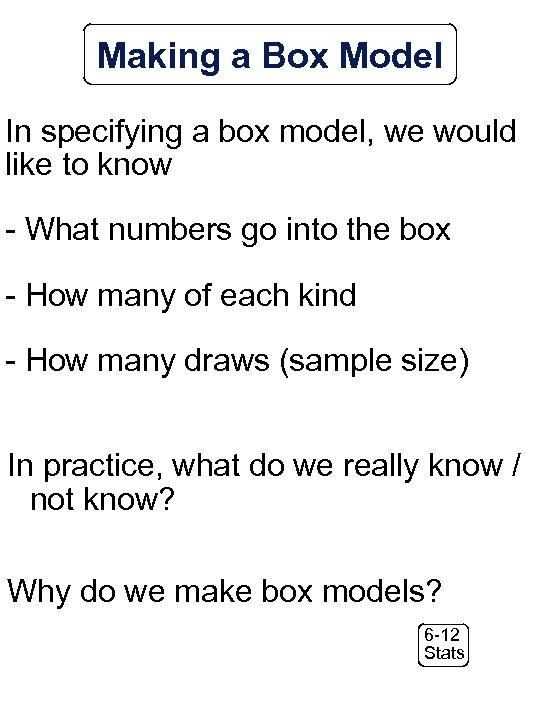Making a Box Model In specifying a box model, we would like to know - What numbers go into the box - How many of each kind - How many draws (sample size) In practice, what do we really know / not know? Why do we make box models? 6 -12 Stats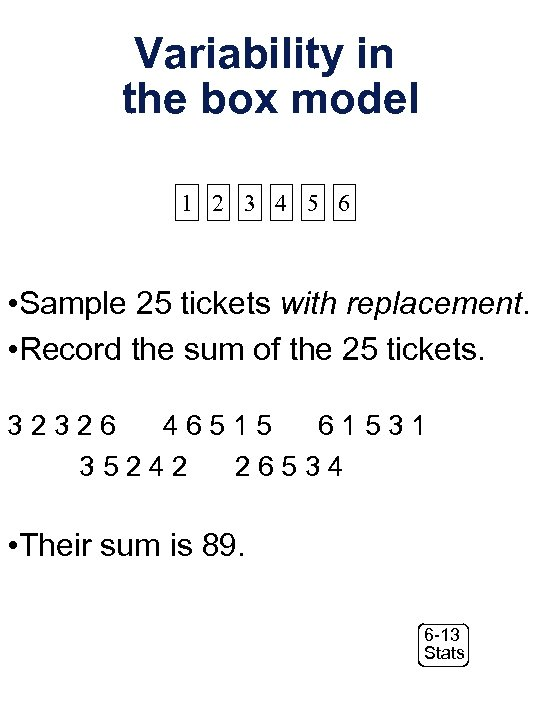Variability in the box model 1 2 3 4 5 6 • Sample 25 tickets with replacement. • Record the sum of the 25 tickets. 32326 46515 61531 35242 26534 • Their sum is 89. 6 -13 Stats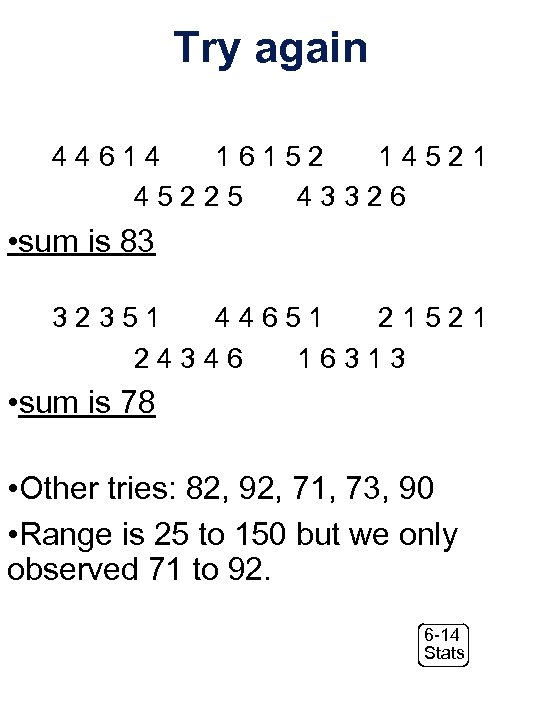Try again 44614 16152 14521 45225 43326 • sum is 83 32351 44651 21521 24346 16313 • sum is 78 • Other tries: 82, 92, 71, 73, 90 • Range is 25 to 150 but we only observed 71 to 92. 6 -14 Stats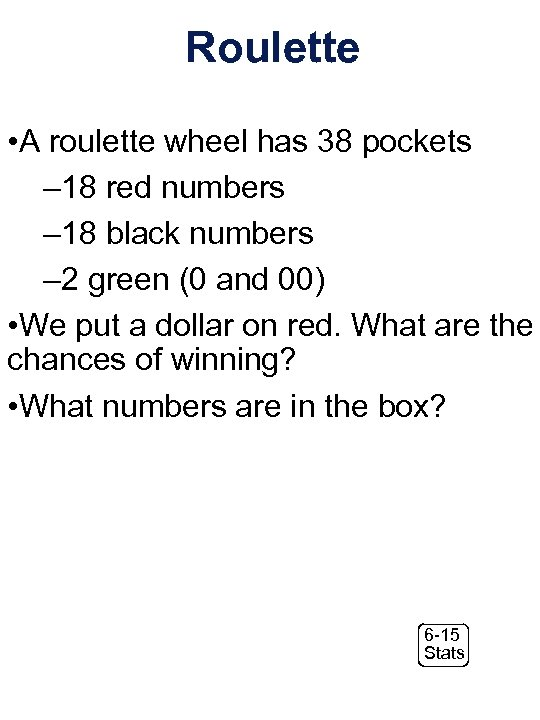Roulette • A roulette wheel has 38 pockets – 18 red numbers – 18 black numbers – 2 green (0 and 00) • We put a dollar on red. What are the chances of winning? • What numbers are in the box? 6 -15 Stats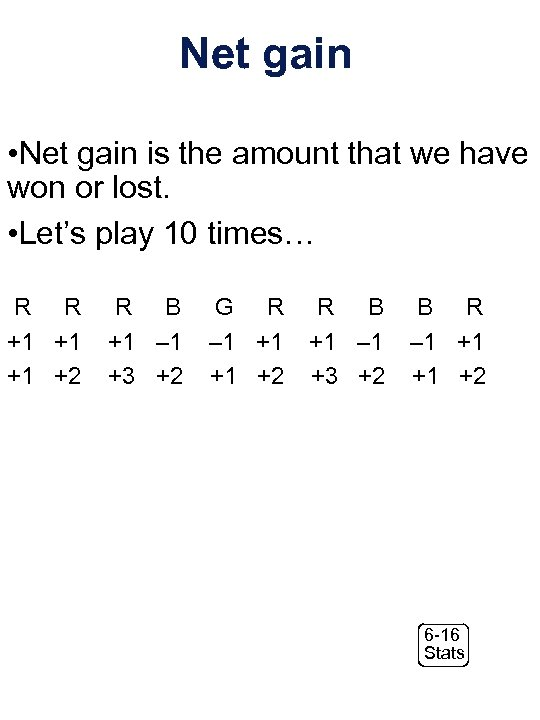Net gain • Net gain is the amount that we have won or lost. • Let’s play 10 times… R R +1 +1 +1 +2 R B +1 – 1 +3 +2 G R – 1 +1 +1 +2 R B +1 – 1 +3 +2 B R – 1 +1 +1 +2 6 -16 StatsSo, Our Box Model is … 6 -17 Stats6 -18 Stats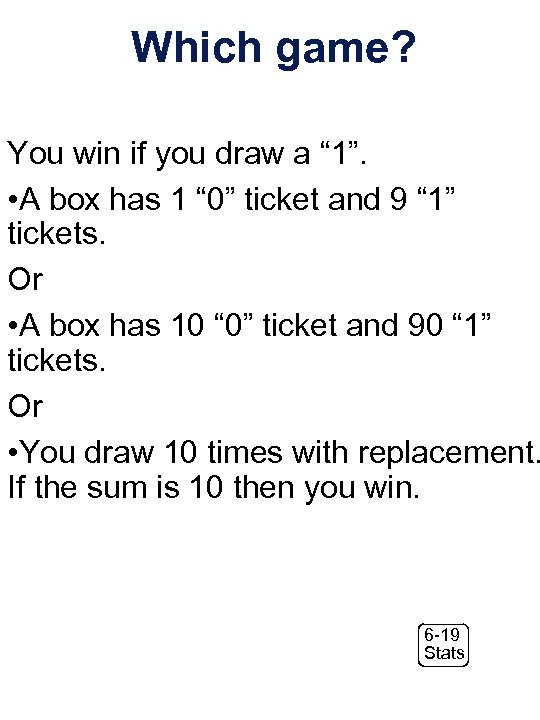Which game? You win if you draw a “ 1”. • A box has 1 “ 0” ticket and 9 “ 1” tickets. Or • A box has 10 “ 0” ticket and 90 “ 1” tickets. Or • You draw 10 times with replacement. If the sum is 10 then you win. 6 -19 StatsOur Box Model is … 6 -20 Stats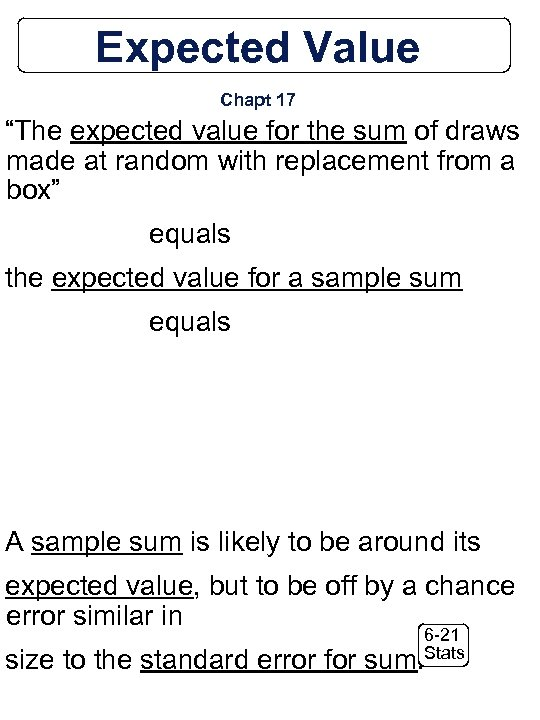Expected Value Chapt 17 “The expected value for the sum of draws made at random with replacement from a box” equals the expected value for a sample sum equals A sample sum is likely to be around its expected value, but to be off by a chance error similar in 6 -21 Stats size to the standard error for sum.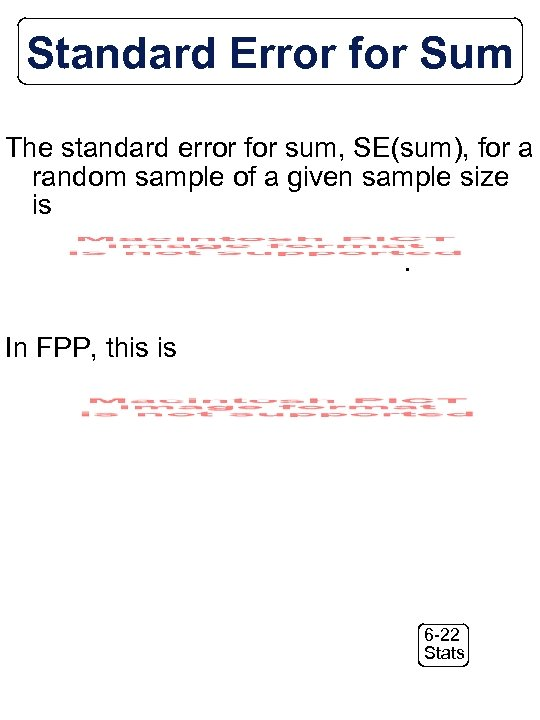Standard Error for Sum The standard error for sum, SE(sum), for a random sample of a given sample size is. In FPP, this is. 6 -22 Stats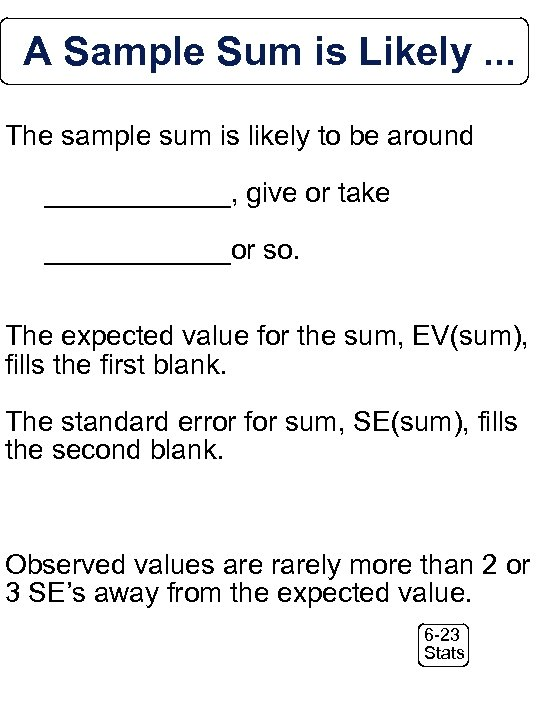A Sample Sum is Likely. . . The sample sum is likely to be around ______, give or take ______or so. The expected value for the sum, EV(sum), fills the first blank. The standard error for sum, SE(sum), fills the second blank. Observed values are rarely more than 2 or 3 SE’s away from the expected value. 6 -23 Stats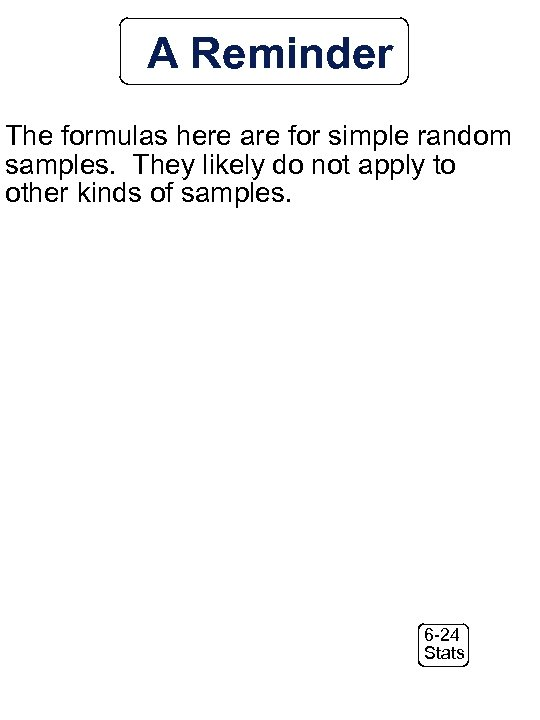A Reminder The formulas here are for simple random samples. They likely do not apply to other kinds of samples. 6 -24 Stats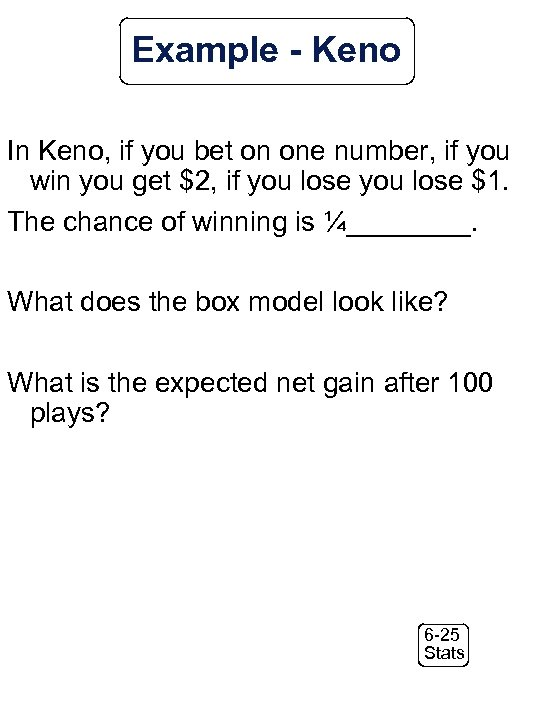Example - Keno In Keno, if you bet on one number, if you win you get \$2, if you lose \$1. The chance of winning is ¼____. What does the box model look like? What is the expected net gain after 100 plays? 6 -25 Stats6 -26 Stats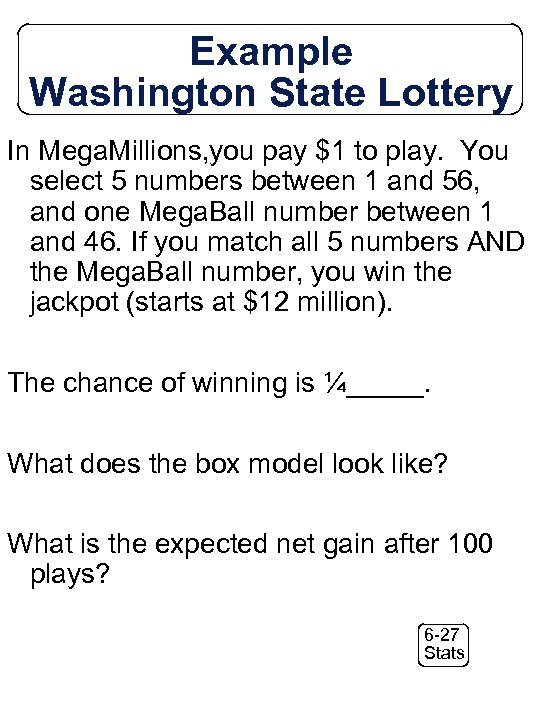Example Washington State Lottery In Mega. Millions, you pay \$1 to play. You select 5 numbers between 1 and 56, and one Mega. Ball number between 1 and 46. If you match all 5 numbers AND the Mega. Ball number, you win the jackpot (starts at \$12 million). The chance of winning is ¼_____. What does the box model look like? What is the expected net gain after 100 plays? 6 -27 Stats6 -28 Stats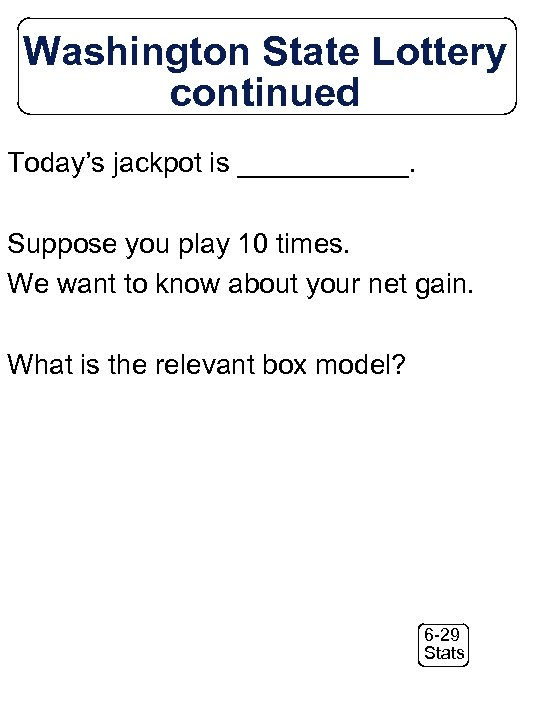Washington State Lottery continued Today’s jackpot is ______. Suppose you play 10 times. We want to know about your net gain. What is the relevant box model? 6 -29 Stats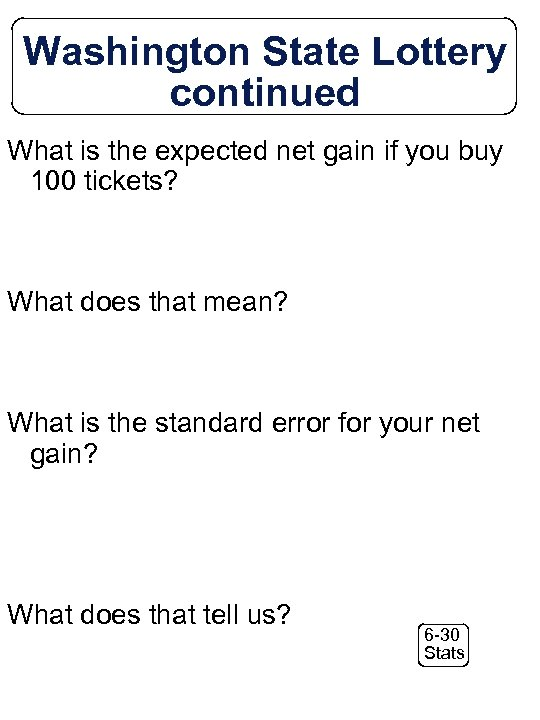Washington State Lottery continued What is the expected net gain if you buy 100 tickets? What does that mean? What is the standard error for your net gain? What does that tell us? 6 -30 Stats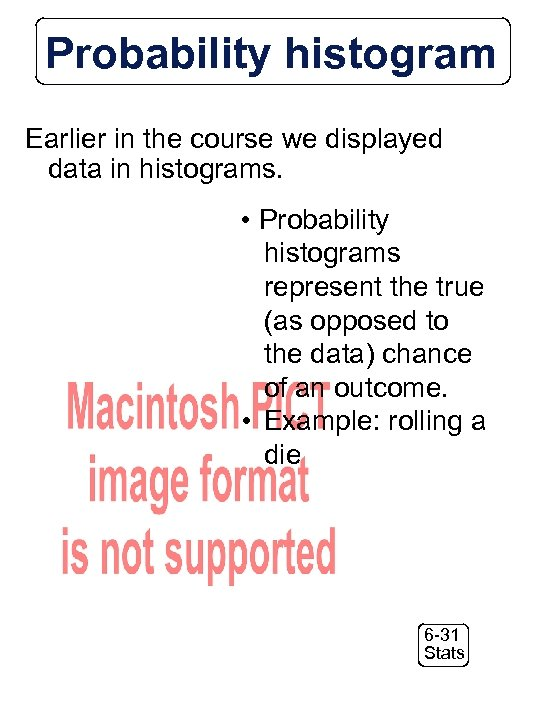Probability histogram Earlier in the course we displayed data in histograms. • Probability histograms represent the true (as opposed to the data) chance of an outcome. • Example: rolling a die 6 -31 Stats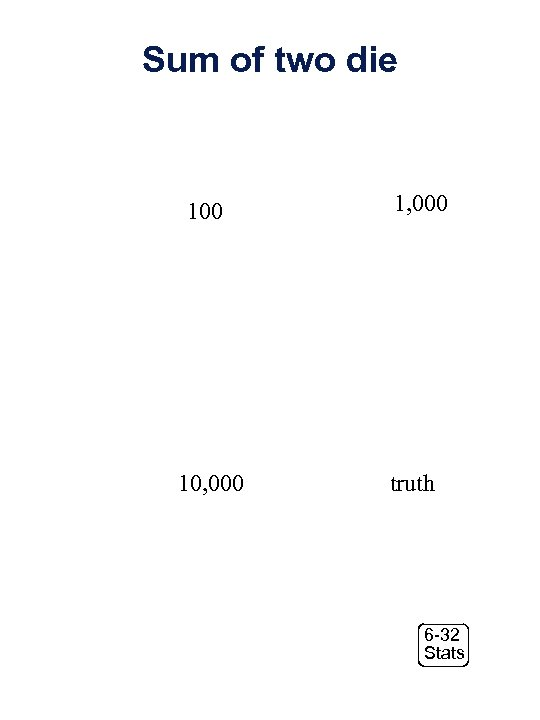Sum of two die 100 10, 000 1, 000 truth 6 -32 Stats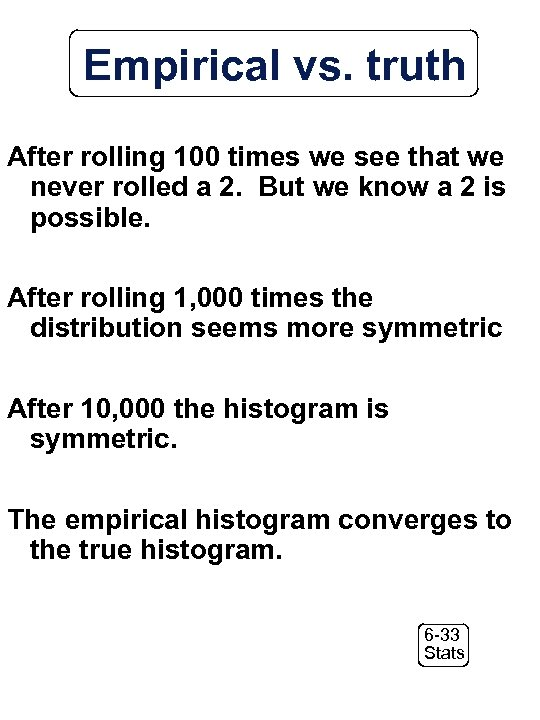Empirical vs. truth After rolling 100 times we see that we never rolled a 2. But we know a 2 is possible. After rolling 1, 000 times the distribution seems more symmetric After 10, 000 the histogram is symmetric. The empirical histogram converges to the true histogram. 6 -33 Stats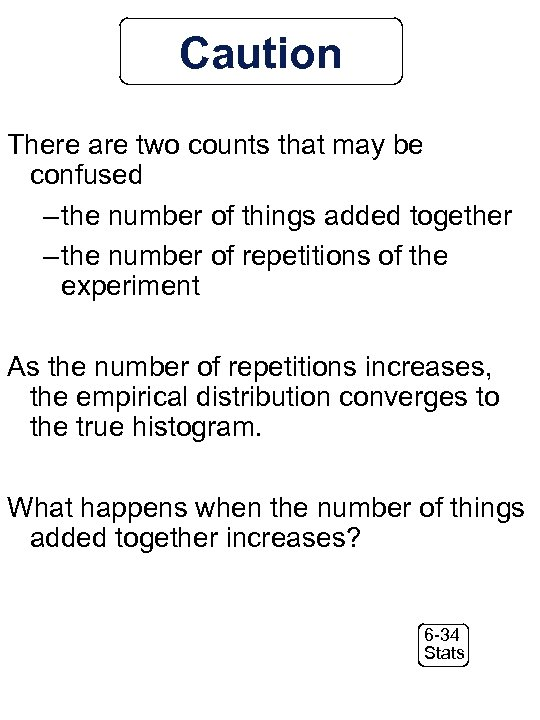Caution There are two counts that may be confused – the number of things added together – the number of repetitions of the experiment As the number of repetitions increases, the empirical distribution converges to the true histogram. What happens when the number of things added together increases? 6 -34 Stats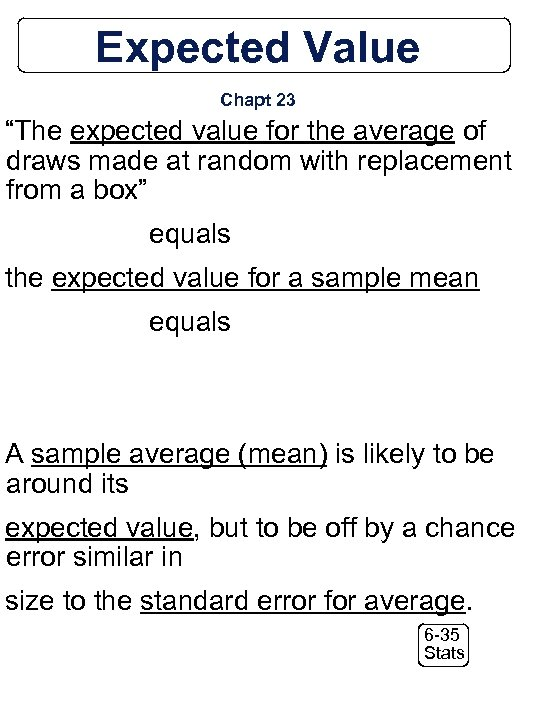Expected Value Chapt 23 “The expected value for the average of draws made at random with replacement from a box” equals the expected value for a sample mean equals A sample average (mean) is likely to be around its expected value, but to be off by a chance error similar in size to the standard error for average. 6 -35 Stats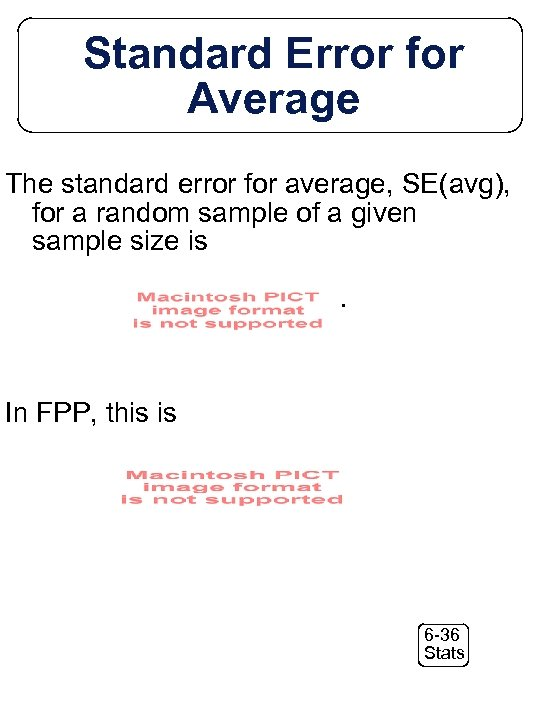Standard Error for Average The standard error for average, SE(avg), for a random sample of a given sample size is. In FPP, this is. 6 -36 Stats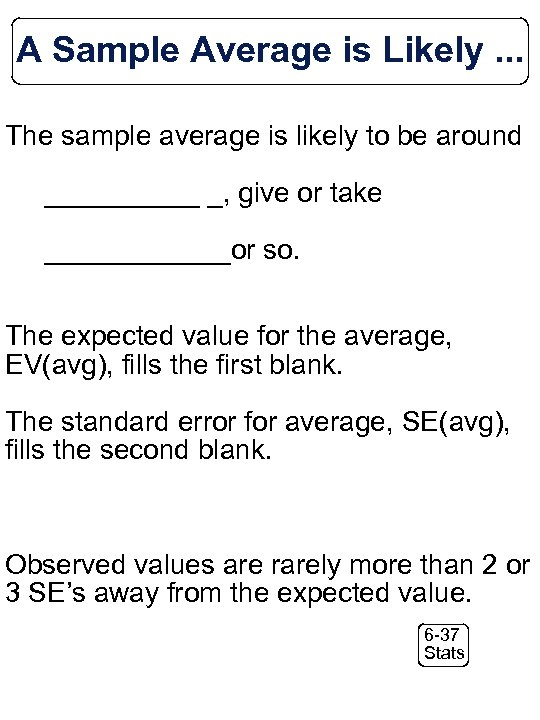A Sample Average is Likely. . . The sample average is likely to be around _____ _, give or take ______or so. The expected value for the average, EV(avg), fills the first blank. The standard error for average, SE(avg), fills the second blank. Observed values are rarely more than 2 or 3 SE’s away from the expected value. 6 -37 Stats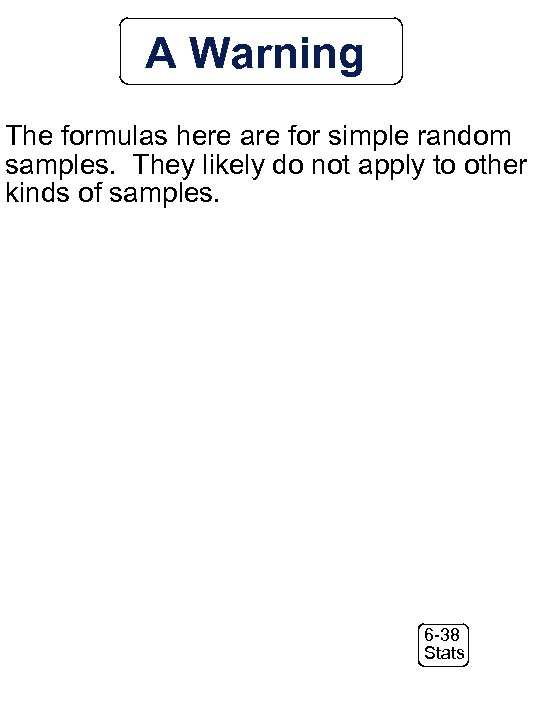A Warning The formulas here are for simple random samples. They likely do not apply to other kinds of samples. 6 -38 Stats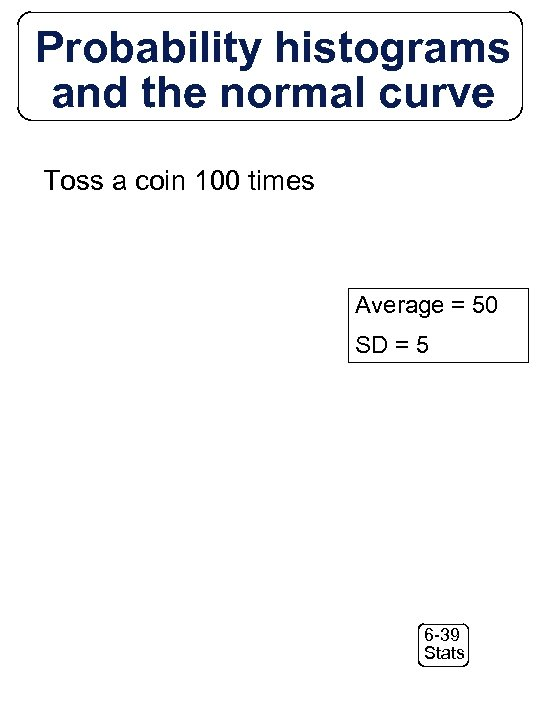Probability histograms and the normal curve Toss a coin 100 times Average = 50 SD = 5 6 -39 Stats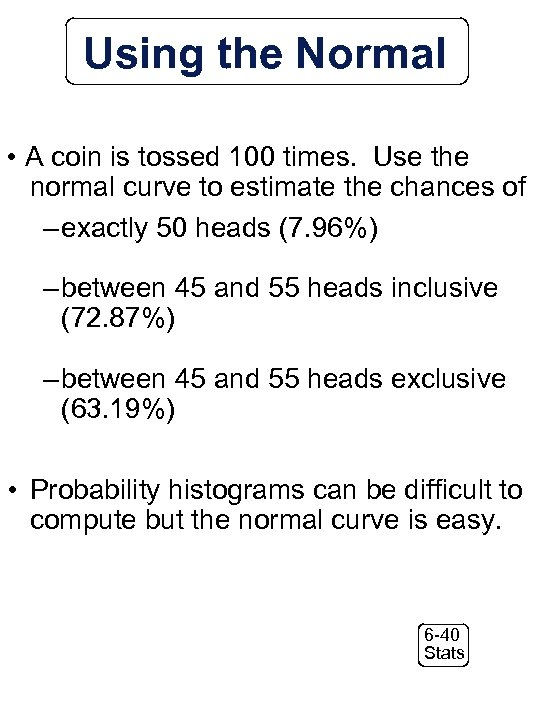Using the Normal • A coin is tossed 100 times. Use the normal curve to estimate the chances of – exactly 50 heads (7. 96%) – between 45 and 55 heads inclusive (72. 87%) – between 45 and 55 heads exclusive (63. 19%) • Probability histograms can be difficult to compute but the normal curve is easy. 6 -40 StatsDrawing from a lopsided box Assume that the box has tickets 1, 9, 5, 5, 5 6 -41 Stats6 -42 Stats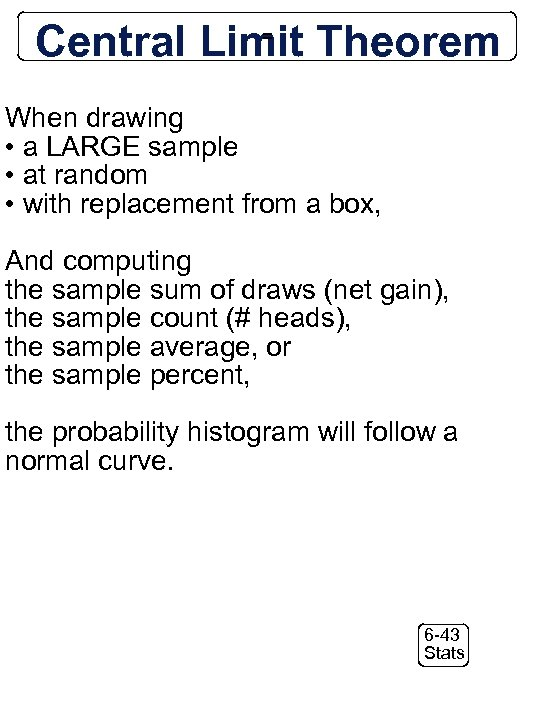Central Limit Theorem = When drawing • a LARGE sample • at random • with replacement from a box, And computing the sample sum of draws (net gain), the sample count (# heads), the sample average, or the sample percent, the probability histogram will follow a normal curve. 6 -43 Stats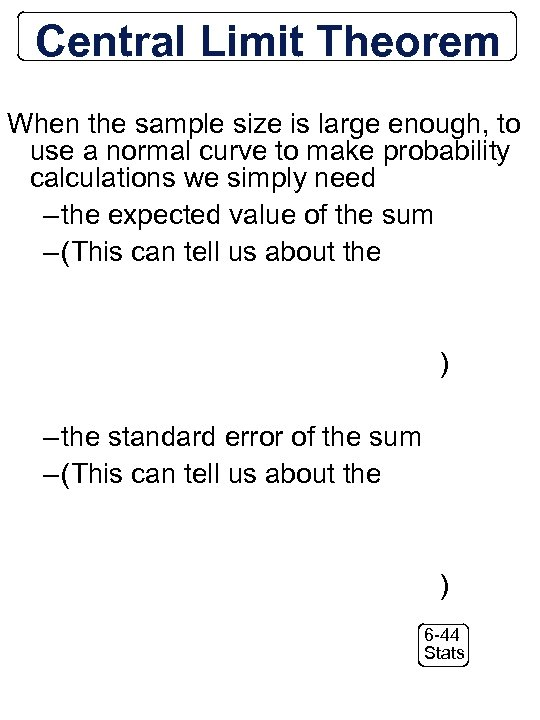Central Limit Theorem When the sample size is large enough, to use a normal curve to make probability calculations we simply need – the expected value of the sum – (This can tell us about the ) – the standard error of the sum – (This can tell us about the ) 6 -44 Stats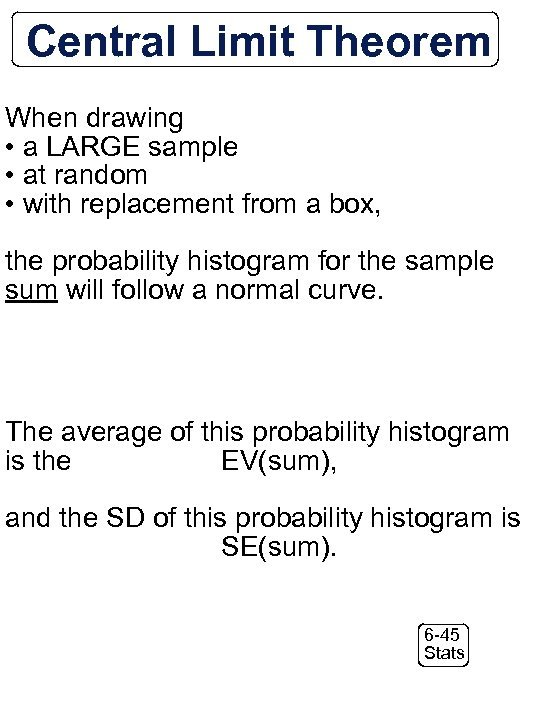Central Limit Theorem When drawing • a LARGE sample • at random • with replacement from a box, the probability histogram for the sample sum will follow a normal curve. The average of this probability histogram is the EV(sum), and the SD of this probability histogram is SE(sum). 6 -45 Stats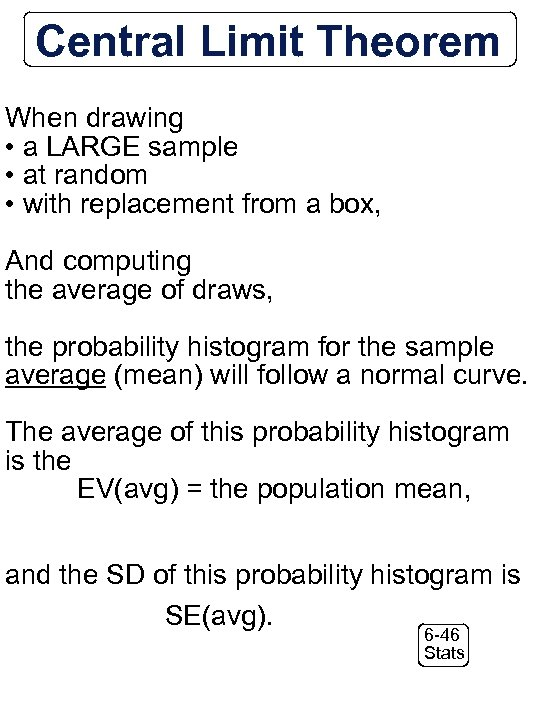Central Limit Theorem When drawing • a LARGE sample • at random • with replacement from a box, And computing the average of draws, the probability histogram for the sample average (mean) will follow a normal curve. The average of this probability histogram is the EV(avg) = the population mean, and the SD of this probability histogram is SE(avg). 6 -46 Stats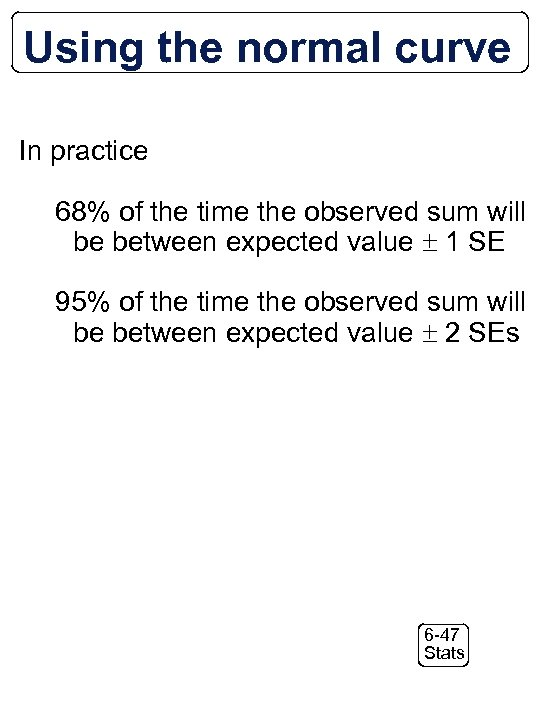Using the normal curve In practice 68% of the time the observed sum will be between expected value 1 SE 95% of the time the observed sum will be between expected value 2 SEs 6 -47 Stats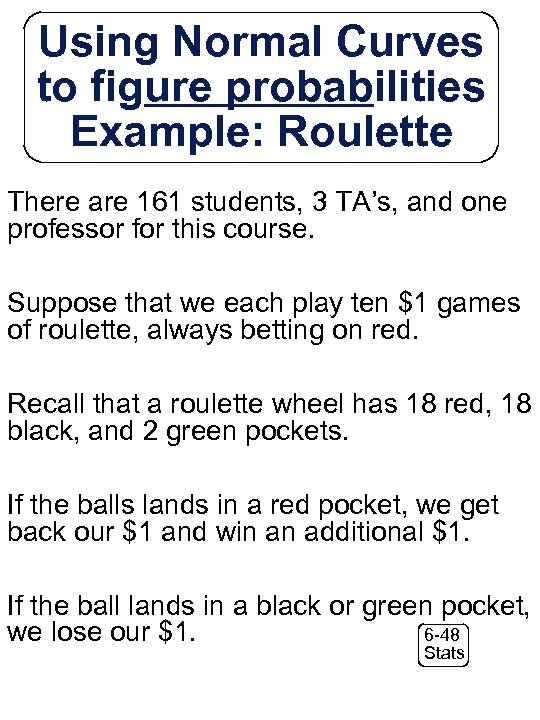Using Normal Curves to figure probabilities Example: Roulette There are 161 students, 3 TA’s, and one professor for this course. Suppose that we each play ten \$1 games of roulette, always betting on red. Recall that a roulette wheel has 18 red, 18 black, and 2 green pockets. If the balls lands in a red pocket, we get back our \$1 and win an additional \$1. If the ball lands in a black or green pocket, 6 -48 we lose our \$1. Stats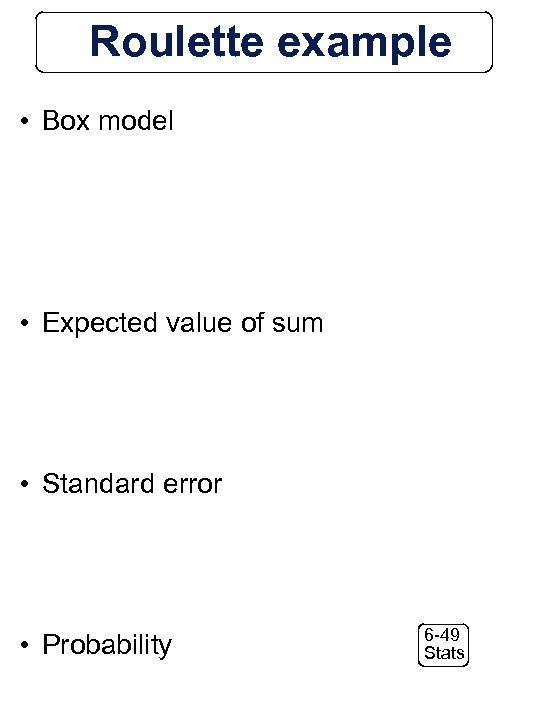Roulette example • Box model • Expected value of sum • Standard error • Probability 6 -49 Stats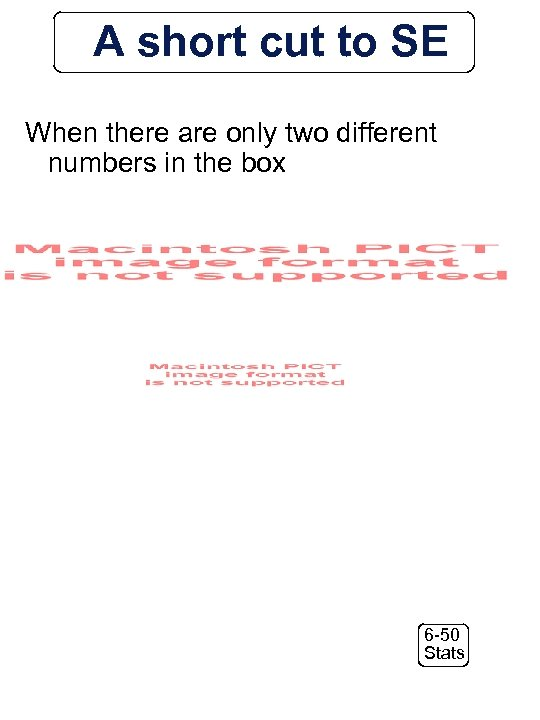A short cut to SE When there are only two different numbers in the box 6 -50 Stats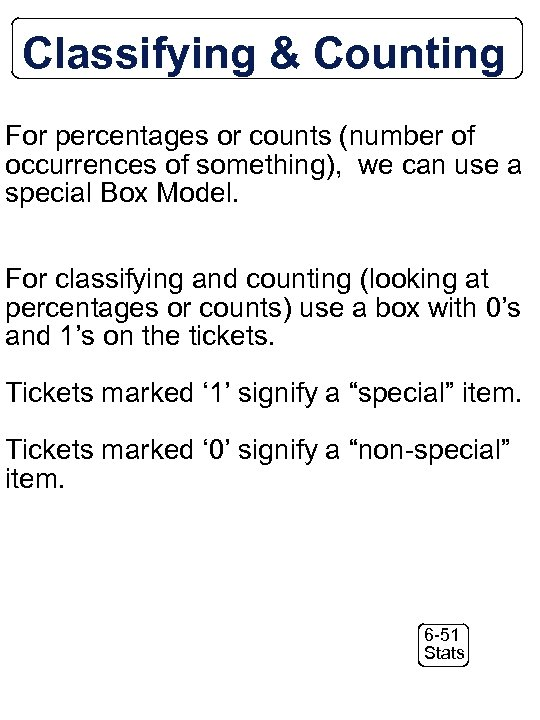Classifying & Counting For percentages or counts (number of occurrences of something), we can use a special Box Model. For classifying and counting (looking at percentages or counts) use a box with 0’s and 1’s on the tickets. Tickets marked ‘ 1’ signify a “special” item. Tickets marked ‘ 0’ signify a “non-special” item. 6 -51 Stats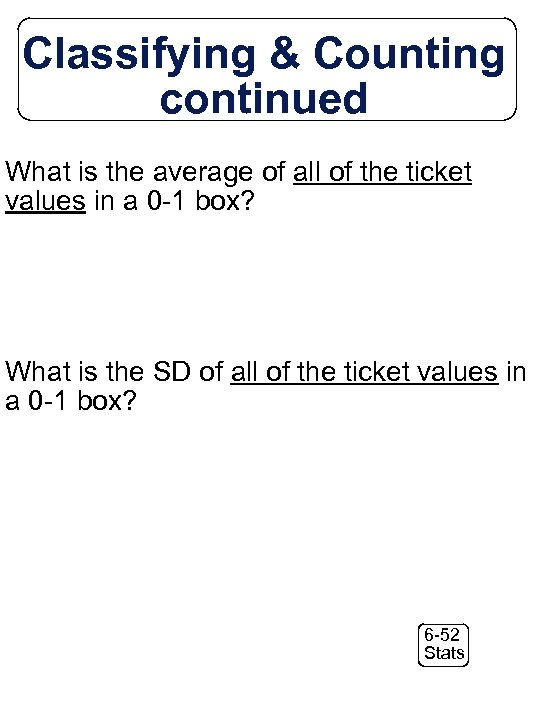Classifying & Counting continued What is the average of all of the ticket values in a 0 -1 box? What is the SD of all of the ticket values in a 0 -1 box? 6 -52 Stats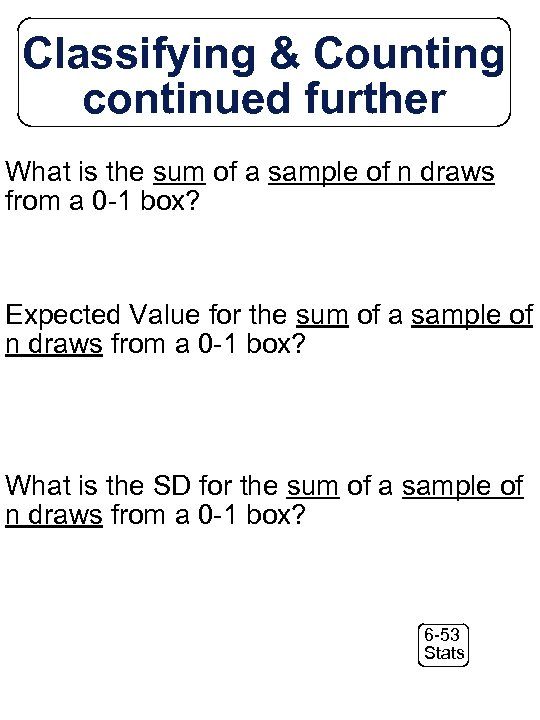Classifying & Counting continued further What is the sum of a sample of n draws from a 0 -1 box? Expected Value for the sum of a sample of n draws from a 0 -1 box? What is the SD for the sum of a sample of n draws from a 0 -1 box? 6 -53 Stats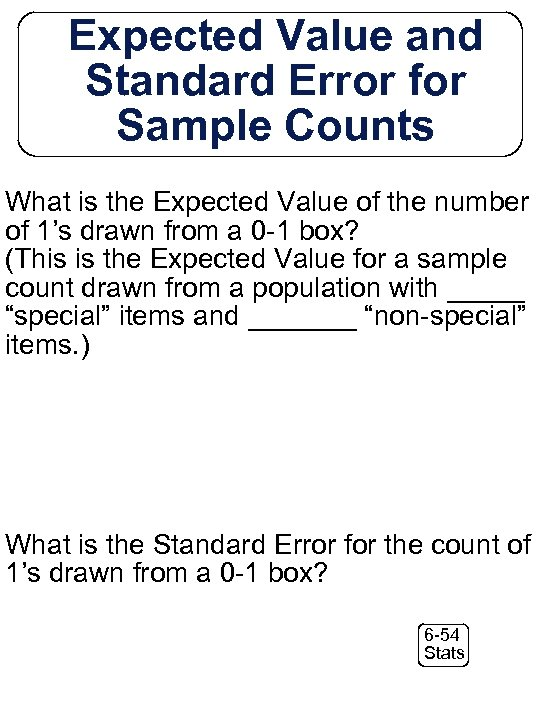Expected Value and Standard Error for Sample Counts What is the Expected Value of the number of 1’s drawn from a 0 -1 box? (This is the Expected Value for a sample count drawn from a population with _____ “special” items and _______ “non-special” items. ) What is the Standard Error for the count of 1’s drawn from a 0 -1 box? 6 -54 Stats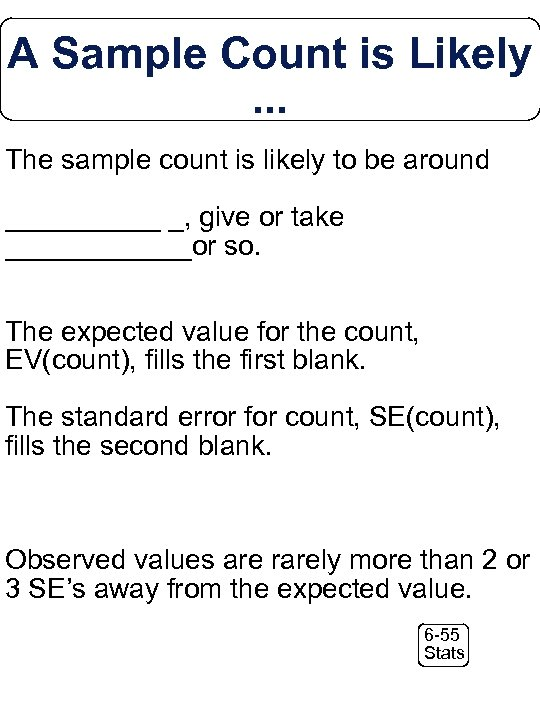A Sample Count is Likely. . . The sample count is likely to be around _____ _, give or take ______or so. The expected value for the count, EV(count), fills the first blank. The standard error for count, SE(count), fills the second blank. Observed values are rarely more than 2 or 3 SE’s away from the expected value. 6 -55 Stats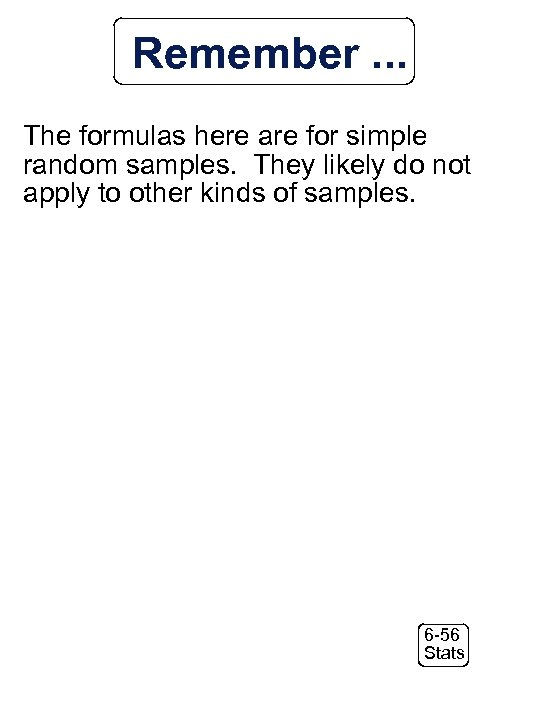Remember. . . The formulas here are for simple random samples. They likely do not apply to other kinds of samples. 6 -56 Stats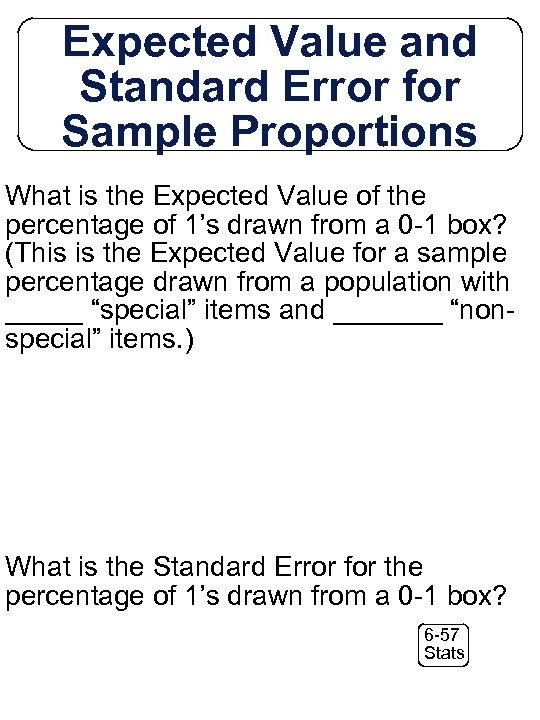Expected Value and Standard Error for Sample Proportions What is the Expected Value of the percentage of 1’s drawn from a 0 -1 box? (This is the Expected Value for a sample percentage drawn from a population with _____ “special” items and _______ “nonspecial” items. ) What is the Standard Error for the percentage of 1’s drawn from a 0 -1 box? 6 -57 Stats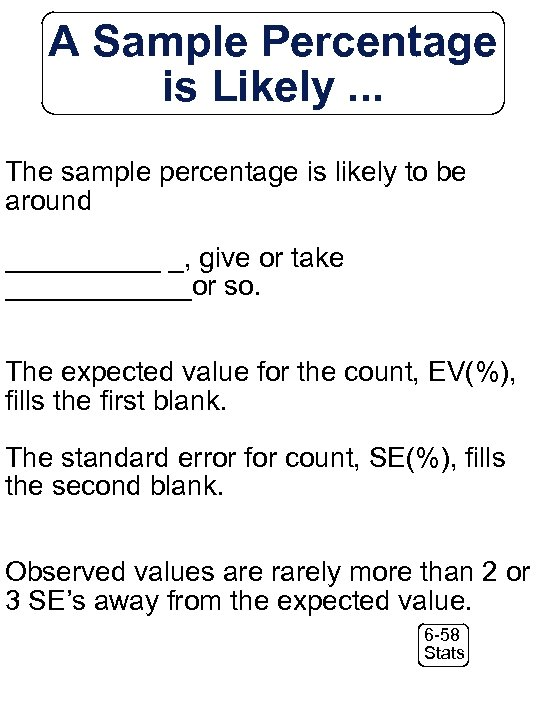A Sample Percentage is Likely. . . The sample percentage is likely to be around _____ _, give or take ______or so. The expected value for the count, EV(%), fills the first blank. The standard error for count, SE(%), fills the second blank. Observed values are rarely more than 2 or 3 SE’s away from the expected value. 6 -58 Stats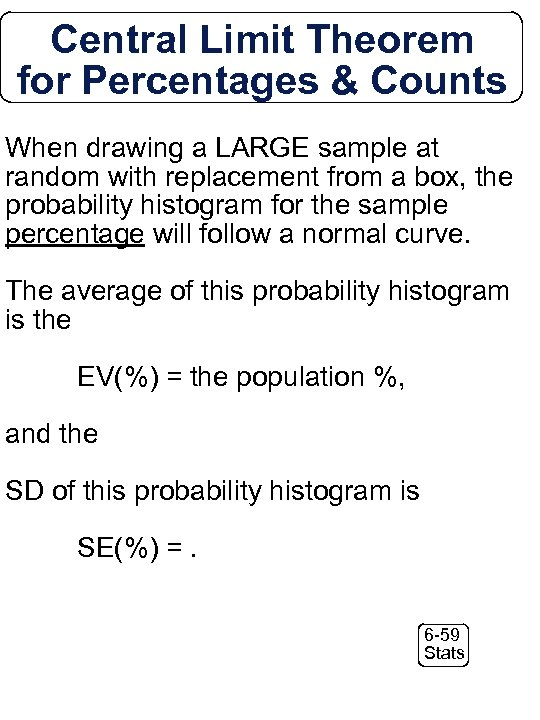Central Limit Theorem for Percentages & Counts When drawing a LARGE sample at random with replacement from a box, the probability histogram for the sample percentage will follow a normal curve. The average of this probability histogram is the EV(%) = the population %, and the SD of this probability histogram is SE(%) =. 6 -59 Stats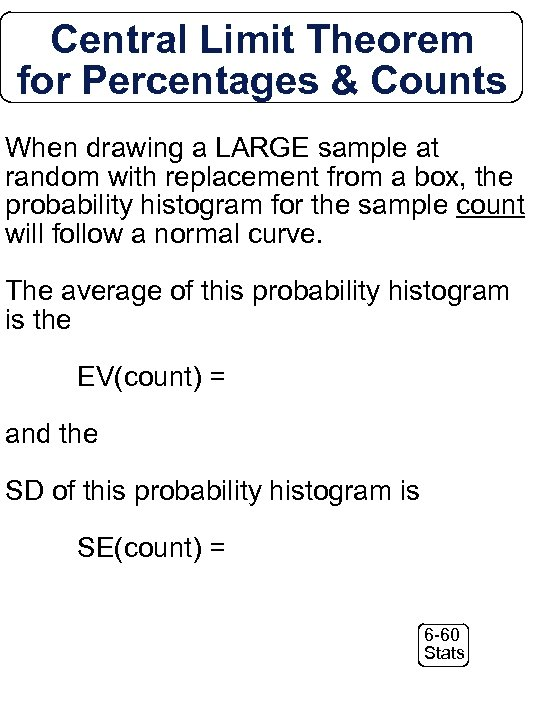Central Limit Theorem for Percentages & Counts When drawing a LARGE sample at random with replacement from a box, the probability histogram for the sample count will follow a normal curve. The average of this probability histogram is the EV(count) = and the SD of this probability histogram is SE(count) = 6 -60 StatsSummarizing … Expected Values and Standard Errors 6 -61 Stats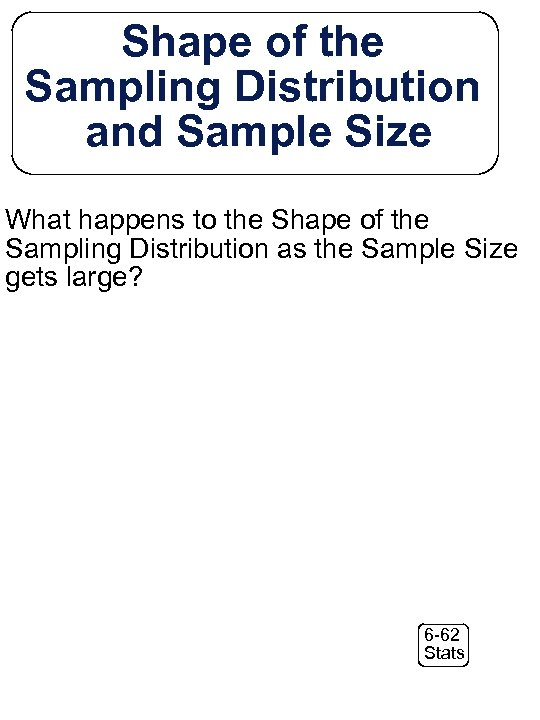Shape of the Sampling Distribution and Sample Size What happens to the Shape of the Sampling Distribution as the Sample Size gets large? 6 -62 Stats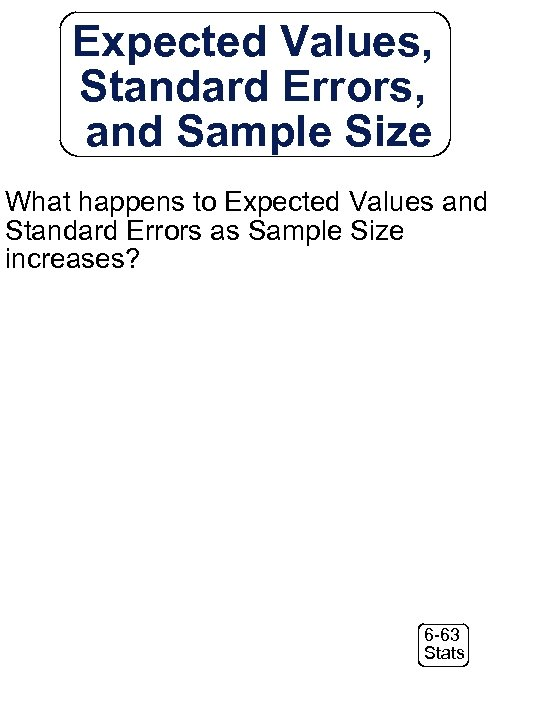Expected Values, Standard Errors, and Sample Size What happens to Expected Values and Standard Errors as Sample Size increases? 6 -63 Stats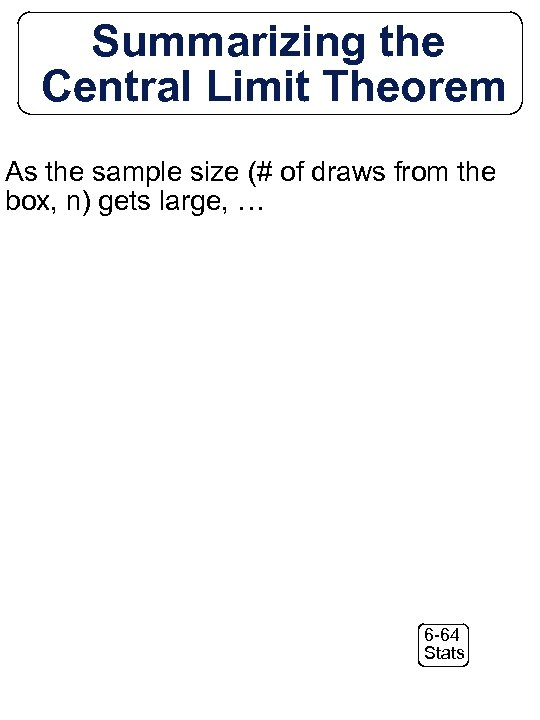Summarizing the Central Limit Theorem As the sample size (# of draws from the box, n) gets large, … 6 -64 Stats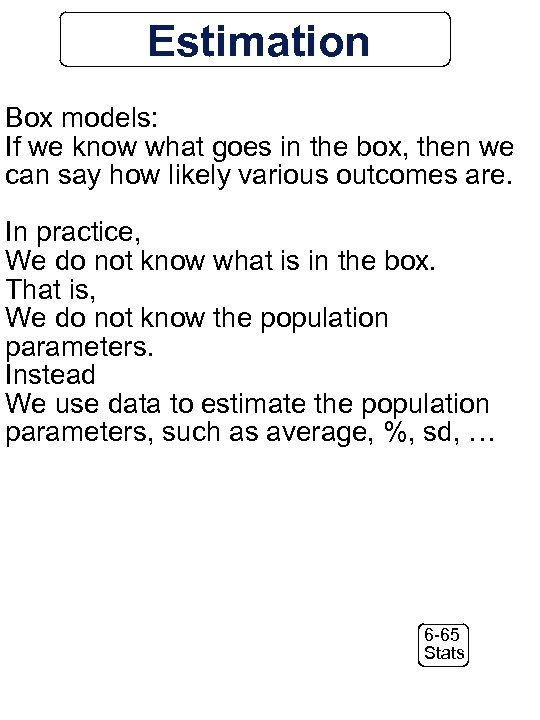Estimation Box models: If we know what goes in the box, then we can say how likely various outcomes are. In practice, We do not know what is in the box. That is, We do not know the population parameters. Instead We use data to estimate the population parameters, such as average, %, sd, … 6 -65 Stats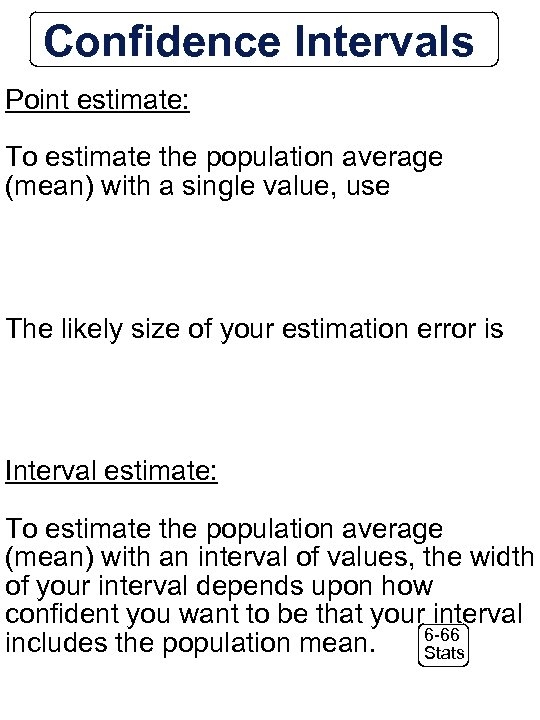Confidence Intervals Point estimate: To estimate the population average (mean) with a single value, use The likely size of your estimation error is Interval estimate: To estimate the population average (mean) with an interval of values, the width of your interval depends upon how confident you want to be that your interval 6 -66 includes the population mean. Stats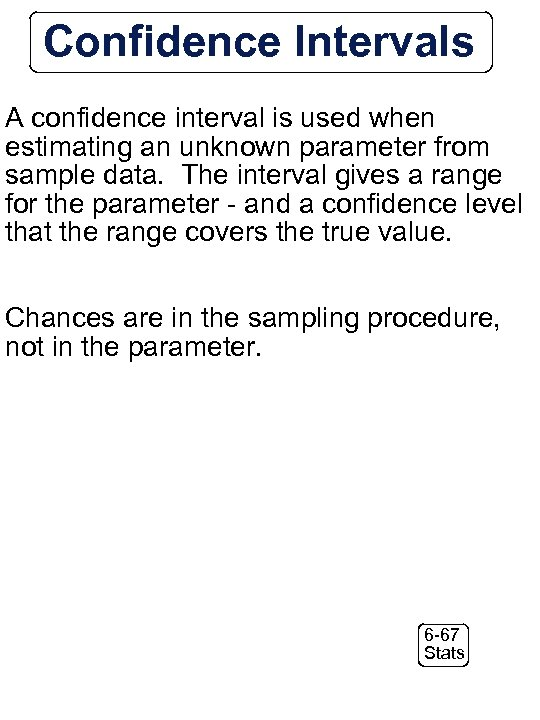Confidence Intervals A confidence interval is used when estimating an unknown parameter from sample data. The interval gives a range for the parameter - and a confidence level that the range covers the true value. Chances are in the sampling procedure, not in the parameter. 6 -67 Stats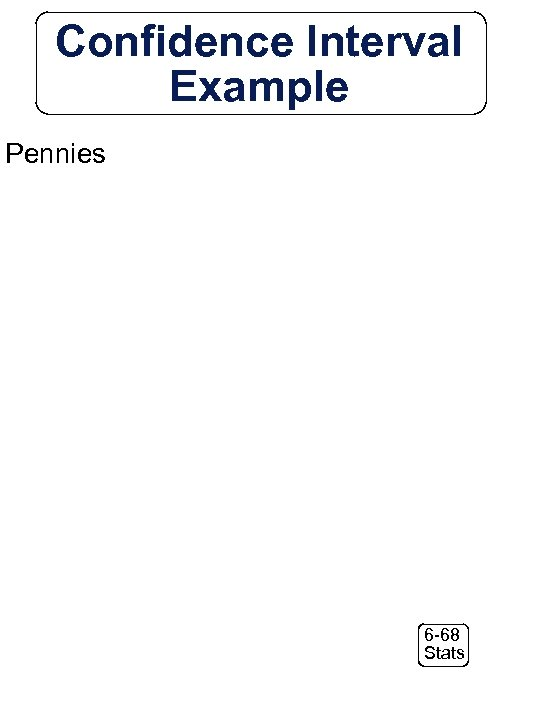Confidence Interval Example Pennies 6 -68 Stats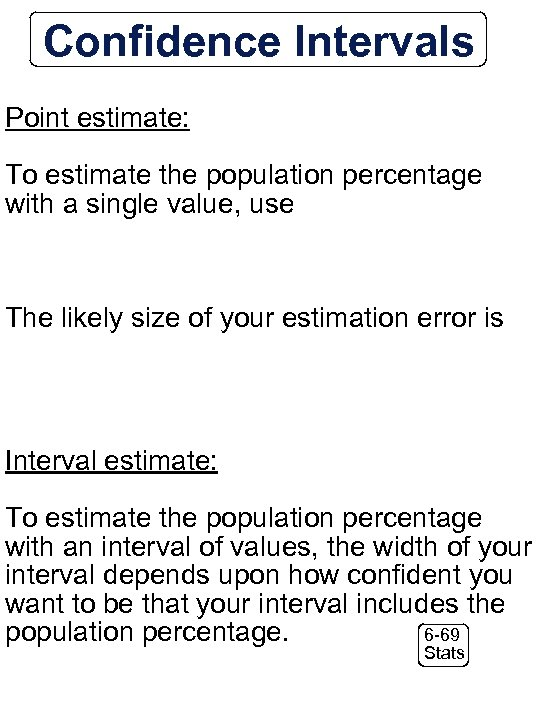Confidence Intervals Point estimate: To estimate the population percentage with a single value, use The likely size of your estimation error is Interval estimate: To estimate the population percentage with an interval of values, the width of your interval depends upon how confident you want to be that your interval includes the 6 -69 population percentage. Stats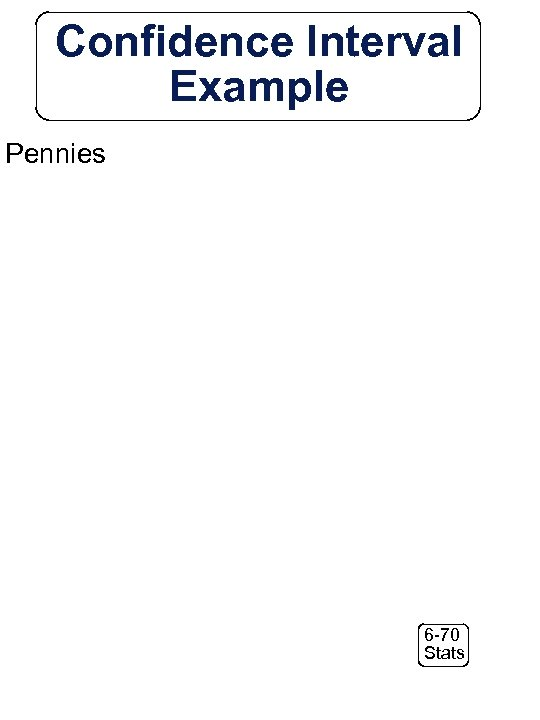Confidence Interval Example Pennies 6 -70 Stats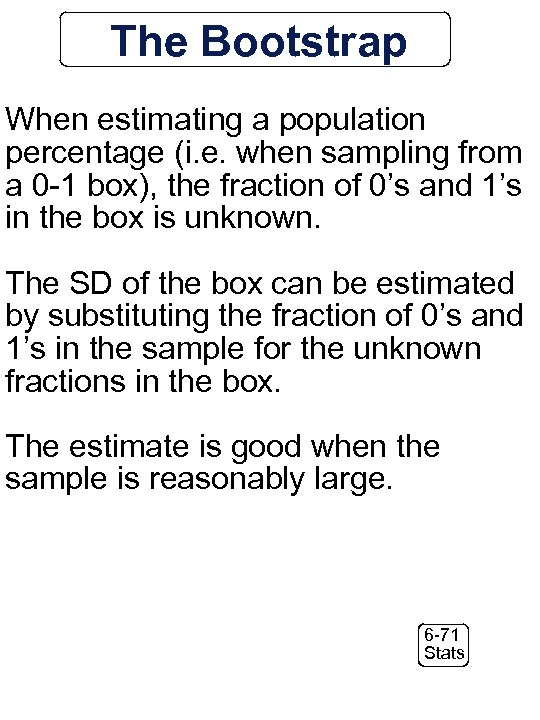The Bootstrap When estimating a population percentage (i. e. when sampling from a 0 -1 box), the fraction of 0’s and 1’s in the box is unknown. The SD of the box can be estimated by substituting the fraction of 0’s and 1’s in the sample for the unknown fractions in the box. The estimate is good when the sample is reasonably large. 6 -71 Stats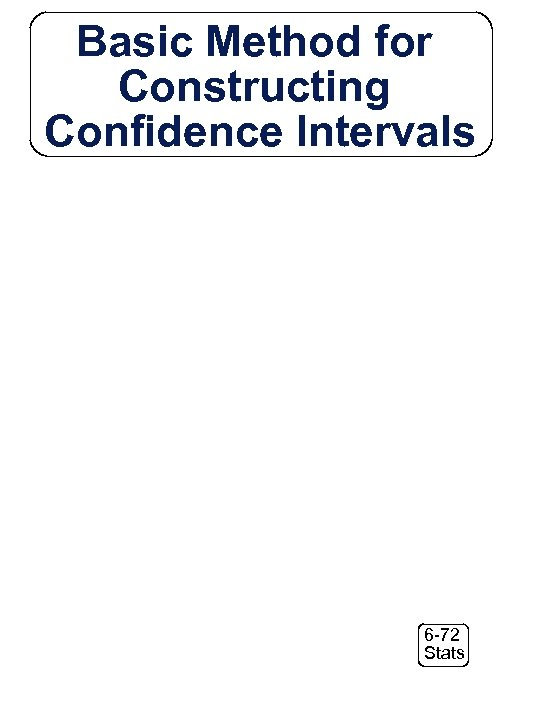Basic Method for Constructing Confidence Intervals 6 -72 Stats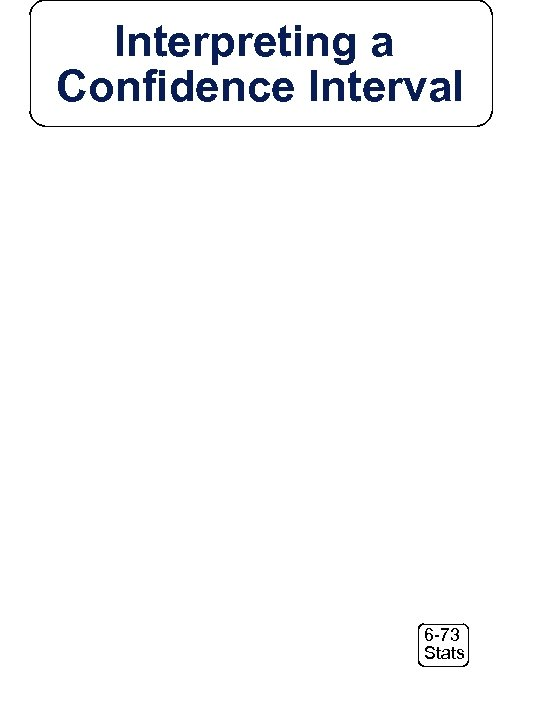Interpreting a Confidence Interval 6 -73 StatsMargin of Error 6 -74 Stats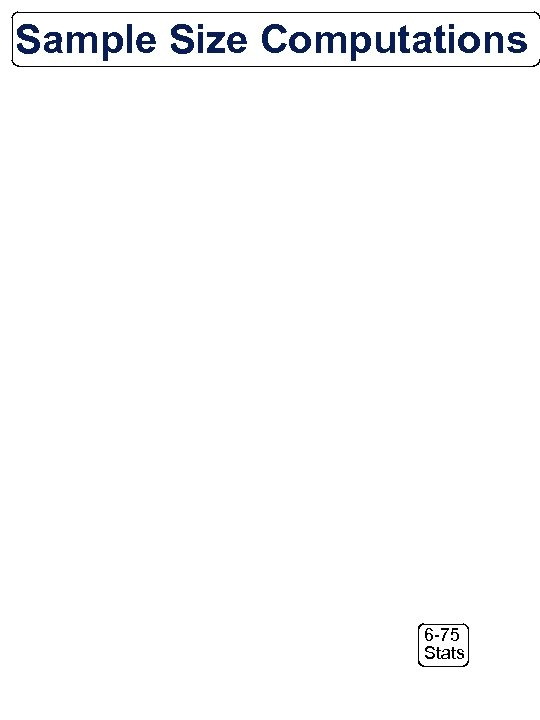Sample Size Computations 6 -75 Stats6 -76 Stats